Next: Is rotation a vector? Up: Rotational motion Previous: Introduction

## Rigid body rotation

Consider a rigid body executing pure rotational motion (i.e., rotational motion which has no translational component). It is possible to define an axis of rotation (which, for the sake of simplicity, is assumed to pass through the body)--this axis corresponds to the straight-line which is the locus of all points inside the body which remain stationary as the body rotates. A general point located inside the body executes circular motion which is centred on the rotation axis, and orientated in the plane perpendicular to this axis. In the following, we tacitly assume that the axis of rotation remains fixed.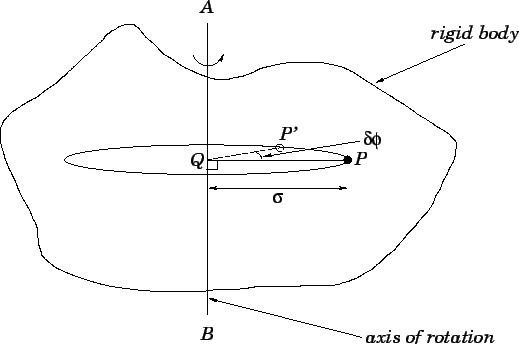Figure 67 shows a typical rigidly rotating body. The axis of rotation is the line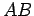. A general point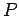lying within the body executes a circular orbit, centred on, in the plane perpendicular to. Let the line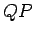be a radius of this orbit which links the axis of rotation to the instantaneous position ofat time. Obviously, this implies thatis normal to. Suppose that at time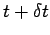pointhas moved to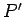, and the radiushas rotated through an angle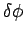. The instantaneous angular velocity of the body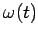is defined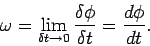(309)

Note that if the body is indeed rotating rigidly, then the calculated value ofshould be the same for all possible pointslying within the body (except for those points lying exactly on the axis of rotation, for whichis ill-defined). The rotation speedof pointis related to the angular velocityof the body via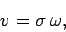(310)

where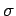is the perpendicular distance from the axis of rotation to point. Thus, in a rigidly rotating body, the rotation speed increases linearly with (perpendicular) distance from the axis of rotation.

It is helpful to introduce the angular acceleration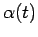of a rigidly rotating body: this quantity is defined as the time derivative of the angular velocity. Thus,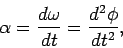(311)

where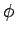is the angular coordinate of some arbitrarily chosen point reference within the body, measured with respect to the rotation axis. Note that angular velocities are conventionally measured in radians per second, whereas angular accelerations are measured in radians per second squared.

For a body rotating with constant angular velocity,, the angular acceleration is zero, and the rotation angleincreases linearly with time: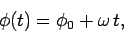(312)

where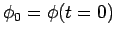. Likewise, for a body rotating with constant angular acceleration,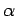, the angular velocity increases linearly with time, so that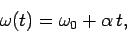(313)

and the rotation angle satisfies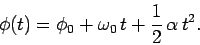(314)

Here,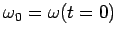. Note that there is a clear analogy between the above equations, and the equations of rectilinear motion at constant acceleration introduced in Sect. 2.6--rotation angle plays the role of displacement, angular velocity plays the role of (regular) velocity, and angular acceleration plays the role of (regular) acceleration.Next: Is rotation a vector? Up: Rotational motion Previous: Introduction
Richard Fitzpatrick 2006-02-02